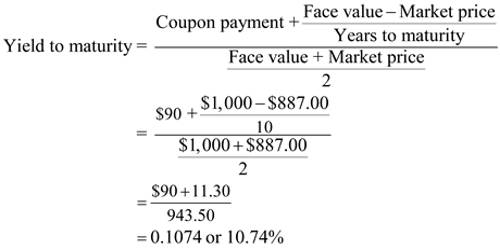# Differentiate between Yield to Maturity (YTM) and Yield to Call (YTC)The yield to maturity (YTM) is that discount rate which causes the present value of the promised payment stream to equal the current price of the bond. It asserts that the bond will be redeemed only at the end of the full maturity period. Yield to Call is the decision to call (or to refund) the issue is the effect of a potential call on a bond’s expected rate of return. If the going rate of interest is well below a callable bond’s coupon rate, then the bond is likely to be called.

Difference between yield to maturity and yield to call: There is some difference between yield to maturity and yield to call. These are followed –

Yield to maturity (YTM)

• YTM is the expected rate of return on a bond if bought at its current market price and to maturity.
• YTM can easily determine using a financial calculator or spreadsheet.
• One must use an initial or error process.
• YTM will riot represent the expected return.
• The effective annual rate of YTM is actually 12.36%.

Yield to call (YTC)

• If the bond can be redeemed before maturity, it is callable, and the return investors will receive it is called is defined as the YTC.
• YTC can more easily determine using a financial calculator or spreadsheet.
• It is not an initial or error process.
• YTC will represent the expected return.
• The effective annual rate of YTC is actually 13.75%.